# (Solved) : Option Explicit Public Card 5 String Rannum 5 Integer Dim Integer Sub Fullhouse Created L Q41340164 . . .

**Using VBA EXCEL**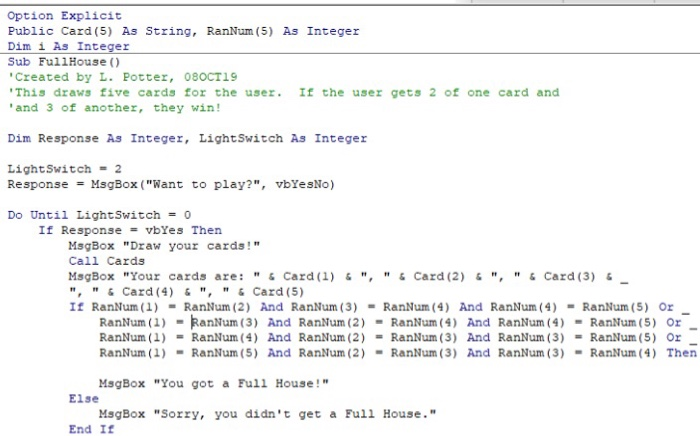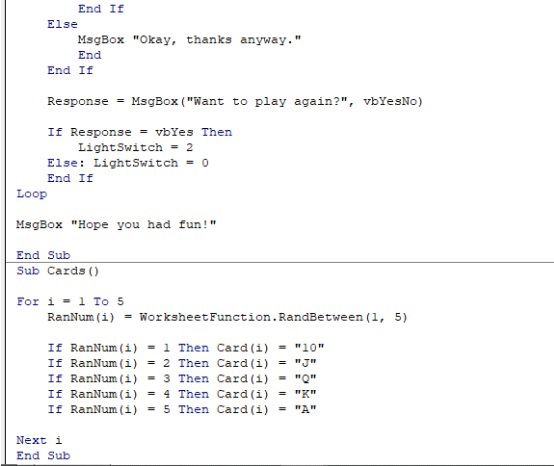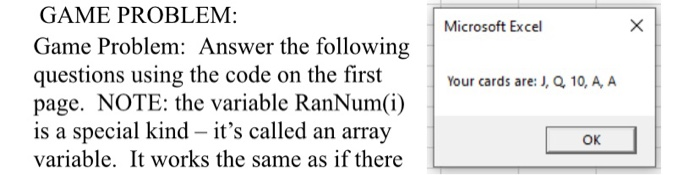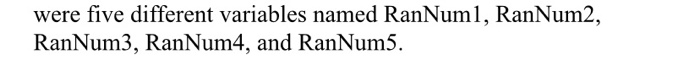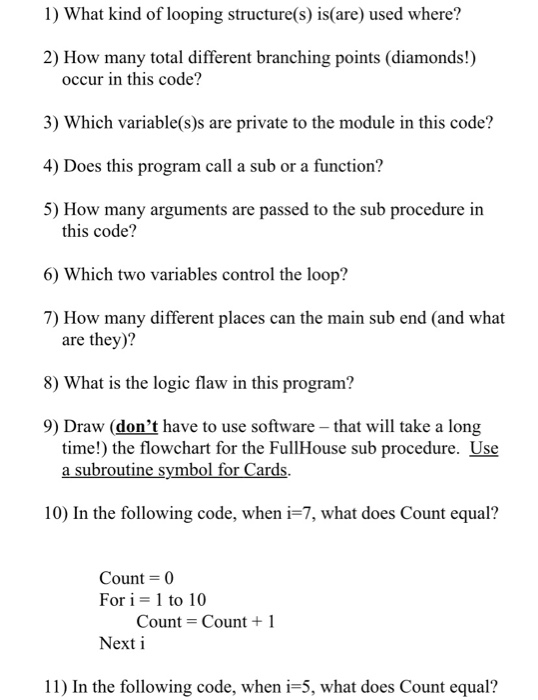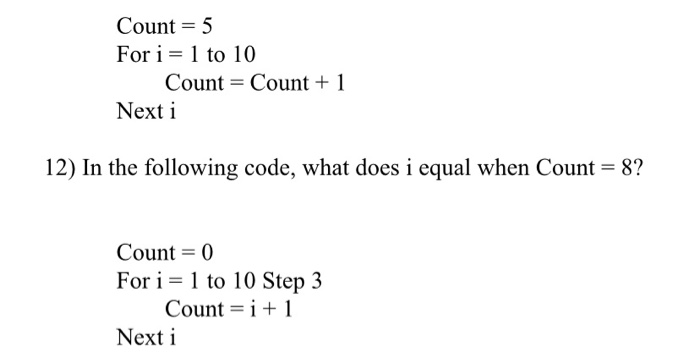Option Explicit Public Card (5) As String, RanNum (5) As Integer Dim i As Integer Sub FullHouse() Created by L. Potter, 080CT19 ‘This draws five cards for the user. If the user gets 2 of one card and and 3 of another, they win! Dim Response As Integer, Light Switch As Integer Light Switch = 2 Response = MsgBox (“Want to play?”, vbYesNo) Do Until Light Switch = 0 If Response = vbYes Then MsgBox “Draw your cards!” Call Cards MsgBox “Your cards are: “& Card (1) 6″, ” & Card (2) 6″, ” & Card (3) 6 “, ” & Card (4) & “, ” & Card (5) If RanNum(1) – RanNum (2) And RanNum (3) – RanNum (4) And RanNum (4) – RanNum (5) Or RanNum(1) – RanNum (3) And RanNum (2) – RanNum (4) And RanNum (4) – RanNum(5) Or RanNum(1) = RanNum (4) And RanNum (2) = RanNum (3) And RanNum (3) = Rannum (5) Or RanNum(1) – RanNum(5) And RanNum (2) – RanNum (3) And RanNum (3) – RanNum (4) Then MsgBox “You got a Full House!” Else MsgBox “Sorry, you didn’t get a Full House.” End If End If Else MsgBox “Okay, thanks anyway.” End End If Response – MsgBox (“Want to play again?”, vbYesNo) If Response = vbYes Then Light Switch = 2 Else: Light Switch = 0 End If Loop MsgBox “Hope you had fun!” End Sub Sub Cards () For i – 1 To 5 RanNum(i) = Worksheet Function. RandBetween(1, 5) If RanNum (1) – 1 Then Card (1) = “10” If RanNum(i) = 2 Then Card (i) = “J” If RanNum (1) – 3 Then Card (i) = “0 If RanNum(i) = 4 Then Card (i) = “K” If RanNum(i) = 5 Then Card (i) = “A” Next i End Sub Microsoft Excel GAME PROBLEM: Game Problem: Answer the following questions using the code on the first page. NOTE: the variable RanNum(i) is a special kind – it’s called an array variable. It works the same as if there Your cards are: J, Q. 10, A, A OK were five different variables named RanNum1, RanNum2, RanNum3, RanNum4, and RanNum5. 1) What kind of looping structure(s) is(are) used where? 2) How many total different branching points (diamonds!) occur in this code? 3) Which variable(s)s are private to the module in this code? 4) Does this program call a sub or a function? 5) How many arguments are passed to the sub procedure in this code? 6) Which two variables control the loop? 7) How many different places can the main sub end (and what are they)? 8) What is the logic flaw in this program? 9) Draw (don’t have to use software – that will take a long time!) the flowchart for the Full House sub procedure. Use a subroutine symbol for Cards. 10) In the following code, when i=7, what does Count equal? Count = 0 For i = 1 to 10 Count = Count +1 Next i 11) In the following code, when i=5, what does Count equal? Count = 5 For i = 1 to 10 Count = Count +1 Next i 12) In the following code, what does i equal when Count = 8? Count = 0 For i = 1 to 10 Step 3 Count = i +1 Next i Show transcribed image text Option Explicit Public Card (5) As String, RanNum (5) As Integer Dim i As Integer Sub FullHouse() Created by L. Potter, 080CT19 ‘This draws five cards for the user. If the user gets 2 of one card and and 3 of another, they win! Dim Response As Integer, Light Switch As Integer Light Switch = 2 Response = MsgBox (“Want to play?”, vbYesNo) Do Until Light Switch = 0 If Response = vbYes Then MsgBox “Draw your cards!” Call Cards MsgBox “Your cards are: “& Card (1) 6″, ” & Card (2) 6″, ” & Card (3) 6 “, ” & Card (4) & “, ” & Card (5) If RanNum(1) – RanNum (2) And RanNum (3) – RanNum (4) And RanNum (4) – RanNum (5) Or RanNum(1) – RanNum (3) And RanNum (2) – RanNum (4) And RanNum (4) – RanNum(5) Or RanNum(1) = RanNum (4) And RanNum (2) = RanNum (3) And RanNum (3) = Rannum (5) Or RanNum(1) – RanNum(5) And RanNum (2) – RanNum (3) And RanNum (3) – RanNum (4) Then MsgBox “You got a Full House!” Else MsgBox “Sorry, you didn’t get a Full House.” End If
End If Else MsgBox “Okay, thanks anyway.” End End If Response – MsgBox (“Want to play again?”, vbYesNo) If Response = vbYes Then Light Switch = 2 Else: Light Switch = 0 End If Loop MsgBox “Hope you had fun!” End Sub Sub Cards () For i – 1 To 5 RanNum(i) = Worksheet Function. RandBetween(1, 5) If RanNum (1) – 1 Then Card (1) = “10” If RanNum(i) = 2 Then Card (i) = “J” If RanNum (1) – 3 Then Card (i) = “0 If RanNum(i) = 4 Then Card (i) = “K” If RanNum(i) = 5 Then Card (i) = “A” Next i End Sub
Microsoft Excel GAME PROBLEM: Game Problem: Answer the following questions using the code on the first page. NOTE: the variable RanNum(i) is a special kind – it’s called an array variable. It works the same as if there Your cards are: J, Q. 10, A, A OK
were five different variables named RanNum1, RanNum2, RanNum3, RanNum4, and RanNum5.
1) What kind of looping structure(s) is(are) used where? 2) How many total different branching points (diamonds!) occur in this code? 3) Which variable(s)s are private to the module in this code? 4) Does this program call a sub or a function? 5) How many arguments are passed to the sub procedure in this code? 6) Which two variables control the loop? 7) How many different places can the main sub end (and what are they)? 8) What is the logic flaw in this program? 9) Draw (don’t have to use software – that will take a long time!) the flowchart for the Full House sub procedure. Use a subroutine symbol for Cards. 10) In the following code, when i=7, what does Count equal? Count = 0 For i = 1 to 10 Count = Count +1 Next i 11) In the following code, when i=5, what does Count equal?
Count = 5 For i = 1 to 10 Count = Count +1 Next i 12) In the following code, what does i equal when Count = 8? Count = 0 For i = 1 to 10 Step 3 Count = i +1 Next i